# Learning Clojure

## Sunday, December 19, 2021

### Fermat's Christmas Theorem: Windmills

```#!/usr/bin/env clojure

;; Fermats' Christmas Theorem: Windmills

;; Sorry for the delay, I had COVID. I'm fine, don't worry!

;; Let's pick an arbitrary number of the form 4n+1, say 29

;; Precisely because it's of form 4n+1, we can split it into a central square and four identical
;; blocks, in this case, a 1x1 square and four 1x7 blocks

;; 29 = 1*1 + 4 * (1 * 7)
(+ (* 1 1) (* 4 (* 1 7))) ; 29

;; Let's draw that:

;; I'm not going to explain how the svg making thing works, but see:
;; http://www.learningclojure.com/2010/10/generating-xml-to-make-svg-vector.html
;; if you're curious about the details

(require 'clojure.xml)

(def squaresize 10)

(defn make-rect [i j colour]
{:tag :rect
:attrs {:x (str (* i squaresize))
:y (str (* j squaresize))
:width  (str squaresize)
:height (str squaresize)
:style (str "fill:", colour, ";stroke:black;stroke-width:1px;stroke-linecap:butt;stroke-linejoin:miter;stroke-opacity:1")}})

;; SVG coordinates have 0,0 in the top left corner, whereas I like my origin in the middle,
;; and with the vertical component increasing as we go up, so this is a coordinate transform
;; That converts my system to SVG's system.
(let [hmin (apply min (map first  rectlist))
vmax (apply max (map second rectlist))]
(for [[a b c] rectlist] [(- a hmin) (- vmax b) c])))

(defn make-svg [objects]
{:tag :svg
:attrs {:width "100%"
:height "100%"
:version "1.1"
:xmlns "http://www.w3.org/2000/svg"}
:content (for [[i j c] (adjust-list objects)] (make-rect i j c))})

(defn svg-file [filename objects]
(spit (str filename ".svg") (with-out-str (clojure.xml/emit (make-svg objects)))))

(defn orange [n]
(if (< n 0)
(range 0 n -1)
(range 0 n 1)))

(defn make-composite-rectangle [h v hsquares vsquares colour]
(for [i (orange hsquares) j (orange vsquares)] [(+ i h) (+ j v) colour]))

;; With this drawing code in hand we can diagram 29 = 1 * 1 + 4 * (1 * 7)

;; As this windmill shape:
(svg-file "windmill-29-1"
(concat (make-composite-rectangle  0  0   1   1 "red")
(make-composite-rectangle  1  0   7   1 "white")
(make-composite-rectangle -1  0  -7  -1 "white")
(make-composite-rectangle  0  1  -1   7 "green")
(make-composite-rectangle  0 -1   1  -7 "green")))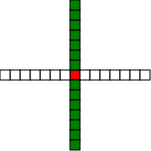;; Or alternatively we could show it as 29 = 1*1 + 4 * (1 * 7)

(svg-file "windmill-29-2"
(concat (make-composite-rectangle  0  0   1   1 "red")
(make-composite-rectangle  1  0   1   7 "white")
(make-composite-rectangle -1  0  -1  -7 "white")
(make-composite-rectangle  0  1  -7   1 "green")
(make-composite-rectangle  0 -1   7  -1 "green")))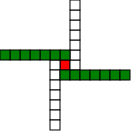;; Now we notice that there's a 3x3 square in the middle, so what about:

(svg-file "windmill-29-3"
(concat (make-composite-rectangle  -1  -1     3   3 "red")
(make-composite-rectangle   1   2     1   5 "white")
(make-composite-rectangle  -1  -2    -1  -5 "white")
(make-composite-rectangle  -2   1    -5   1 "green")
(make-composite-rectangle   2  -1     5  -1 "green")))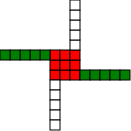;; Which is equivalent to 29 = 3*3 + 4* (1 * 5)

;; And then of course we can flatten the arms again:

;; 29 = 3*3 + 4 * ( 5 * 1 )

(svg-file "windmill-29-4"
(concat (make-composite-rectangle  -1  -1   3   3 "red")
(make-composite-rectangle   1   2  -5   1 "white")
(make-composite-rectangle  -1  -2   5  -1 "white")
(make-composite-rectangle  -2   1   1  -5 "green")
(make-composite-rectangle   2  -1   1   5 "green")))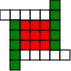;; And we see a 5*5 square now, so

;; 29 = 5*5 + 4 * ( 1 * 1)

(svg-file "windmill-29-5"
(concat (make-composite-rectangle  -2  -2   5   5 "red")
(make-composite-rectangle  -3   2  -1   1 "white")
(make-composite-rectangle   3  -2   1   1 "white")
(make-composite-rectangle  -2  -3   1  -1 "green")
(make-composite-rectangle   2   3   1   1 "green")))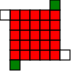;; Notice now that our diagram is one big odd square, and four little squares

;; Four little squares can be combined into one big even square

;; 4*1*1 is also equal to 2*2*1*1, =  (2*1)*(2*1) = 2*2

;; so 29 = 5*5+2*2

;; Which is to say that 29, a prime number of form 4n + 1, is equal to the sum of an odd and an even square.

;; 29 = 25 + 4

;; These windmill drawings form the core of the proof of the Christmas Theorem. Try the technique out on some other numbers!
```